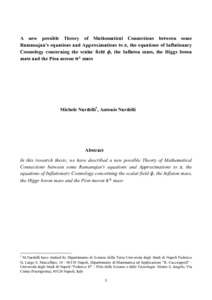# A new possible Theory of Mathematical Connections between some Ramanujan's equations and Approximations to π, the equations of Inflationary Cosmology concerning the scalar field

Nardelli, Michele A new possible Theory of Mathematical Connections between some Ramanujan's equations and Approximations to π, the equations of Inflationary Cosmology concerning the scalar field. Number Theory. (Unpublished)Preview
Text
manard57.pdf - Accepted VersionView Item